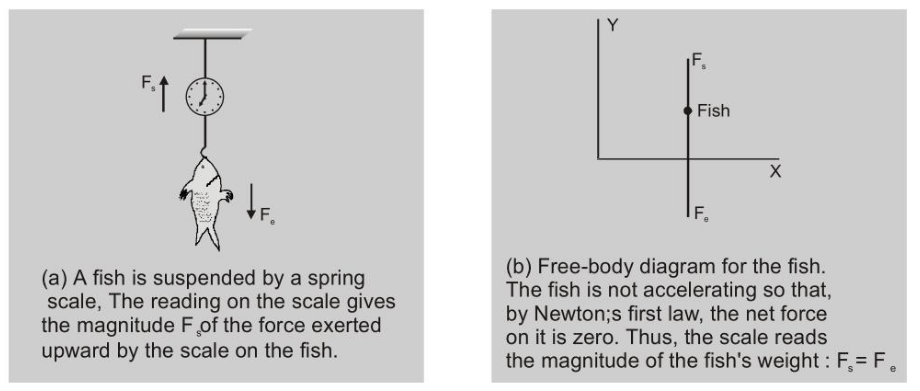Most Affordable JEE | NEET | 8,9,10 Preparation by Kota's Top IITian Doctor Faculties

# What is Newton’s First Law of Motion?## Newton’s First Law of Motion

Newton's first law of motion states that -

A body remains in the state of rest or uniform motion in a straight line unless and until an external force acts on it.

Newton's I law gives the qualitative definition of force, according to which "force is that external cause which tends to change or actually change the state of motion of the body".

Putting Newton's $1^{\text {st }}$ law of motion in simple words, a body will not start moving until and unless an external force acts on it. Once it is set in motion, it will not stop or change its velocity until and unless some force acts upon it once more. The first law of motion is sometimes also known as the law of inertia.

-Inertia is that property of body owing to which the body opposes any change in its state of motion.

-Inertia $\propto$ mass.

There are two conditions on which the $1^{\text {st }}$ law of motion is dependent:

• Objects at rest: When an object is at rest velocity $(\mathrm{v}=0)$ and acceleration $(\mathrm{a}=0)$ are zero. Therefore, the object continues to be at rest.
• Objects in motion: When an object is in motion, velocity is not equal to zero $(v \neq 0)$ while acceleration $(a=$ $0)$ is equal to zero. Therefore, the object will continue to be in motion with constant velocity and in the same direction.
A common application of Newton's first law is the weighing of an object:Fig. (a) shows a fish suspended from a spring scale. In the figure (b), force vectors are used to represent the forces exerted on the fish. The fish's weight $F_{e}$ is the gravitational force exerted on it by the earth (directed downward) and $F_{s}$ is the force exerted by the scale (directed upward).